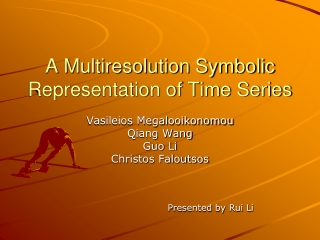DownloadDownload PresentationA Multiresolution Symbolic Representation of Time Series

# A Multiresolution Symbolic Representation of Time Series

Télécharger la présentation## A Multiresolution Symbolic Representation of Time Series

- - - - - - - - - - - - - - - - - - - - - - - - - - - E N D - - - - - - - - - - - - - - - - - - - - - - - - - - -
##### Presentation Transcript

1. A Multiresolution Symbolic Representation of Time Series Vasileios Megalooikonomou Qiang Wang Guo Li Christos Faloutsos Presented by Rui Li

2. Abstract • Introducing a new representation of time series, the Multiresolution Vector Quantized (MVQ) approximation • MVQ keeps both local and global information about the original time series in a hierarchical mechanism • Processing the original time series at multiple resolutions

3. Abstract (cont.) • Representation of time series is symbolic employing key subsequences and potentially allows the application of text-based retrieval techniques into the similarity analysis of time series.

4. Introduction • Two series should be considered similar if they have enough non-overlapping time-ordered pairs of subsequences that are similar.

5. Introduction (cont.) • Instead of calculating the Euclidean distance, first extract key subsequences utilizing the Vector Quantization (VQ) technique and encode each time series based on the frequency of appearance of each key subsequence. • Then calculate similarities in terms of key subsequence matches.

6. Introduction (cont.) • Hierarchical mechanism: the original time series are processed at several different resolutions, and similarity analysis is performed using a weighted distance function combining all the resolution levels

7. Background • Many of the previous work focus on the avoidance of false dismissals. However, in some cases the existence of too many false alarms may decrease the efficiency of retrieval. • The Euclidean distance is not always the optimal distance measure.

8. Background (cont.) • For large datasets, the computational complexity associated with the Euclidean distance calculation is a problem ( O(N*n) ). • Euclidean distance (point-based model) is vulnerable to shape transformations such as shifting and scaling.

9. Background (cont.) • A new framework that utilizes high-level features is proposed • Codebook generation • Time series encoding • Time series representation and retrieval • In order to keep both local and global information, use multiple codebooks with different resolutions

10. Background (cont.) • For each resolution, VQ is applied to discover the vocabulary of subsequences (codewords) • In VQ, a codeword is used to represent a number of similar vectors. • The Generalized Lloyd Algorithm is used to produce a “locally optimal” codebook from a training set.

11. Background (cont.) • To quantitatively measure the similarity between different time series encoded with a VQ codebook, the Histogram Model is employed. • where • and refer to the appearance frequency of codeword in time series t and q, respectively.

12. Proposed Method • MVQ approximation • Partitions each time series into equi-length segments and represents each segment with the most similar key subsequence from a codebook. • Represent each time series as the appearance frequency of each codeword in it. • Apply at several resolutions

13. Proposed Method (cont.) • Codebook Generation • The dataset is preprocessed • Each time series is partitioned into a number of segments each of length l, and each segment forms a sample of the training set that is used to generate the codebook. • Each codeword corresponds to a key subsequence

14. Example1 • Codewords of a 2-level codebook

15. Proposed Method (cont.) • Time Series Encoding • Every time series is decomposed into segments of length l. • For each segment, the closest codeword in the codebook is found and the corresponding index is used to represent this segment. • The appearance frequency of each codeword is counted.

16. Proposed Method (cont.) • Time Series Encoding (cont.) • The representation of a time series is a vector showing the appearance frequency of every codeword.

17. Proposed Method (cont.) • Time Series Summarization • The codewords stand for the most representative subsequences for the entire dataset. • We can just check the appearance frequencies of the codewords and get an overview of the time series.

18. Example2

19. Proposed Method (cont.) • Distance Measure and Multiresolution Representation • Using only one codebook (single resolution) introduces problems • The order among the indices of codewords is not kept; some important global information is lost • Increasing false alarms

20. Proposed Method (cont.) • Distance Measure and Multiresolution Representation (cont.) • A hierarchical mechanism is introduced. • Several different resolutions are involved. • higher resolution → local information lower resolution → global information

21. Example3 • Reconstruction of time series using different resolutions

22. Proposed Method (cont.) • Distance Measure and Multiresolution Representation (cont.) • By being assigned different weights to different resolutions, a weighted similarity measure (Hierarchical Histogram Model) is defined:

23. Experiments • Best Matches Retrieval • SYNDATA • 6 classes; 100 time series for each class; 60 points for each time series

24. Experiments (cont.) • Best Matches Retrieval(cont.) • CAMMOUSE • 1600 points for each time series

25. Experiments (cont.) • Best Matches Retrieval(cont.) • Comparisons with other methods

26. Experiments (cont.) • Clustering • SYNDATA

27. Experiments (cont.) • Clustering (cont.) • CAMMOUSE

28. Thank you!